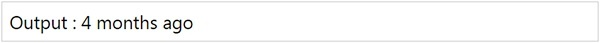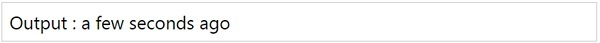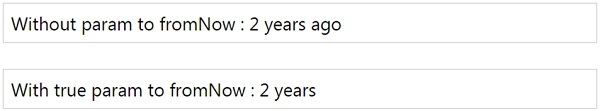# MomentJS - Time from now

This method will tell the length of time from now. Suppose for example if you pass a date to this method it will display the difference if it is in years, months, hours, minutes, or seconds.

## Syntax

```moment().fromNow();
moment().fromNow(Boolean);
```

## Example

```var changeddate = moment([2018, 0, 1]).fromNow();
```

## OutputObserve that the date given to the moment is 01/01/2018, the difference till date is 4 months, so the output is given as 4 months ago.

Observe the following example to check the output when no inputs are given to the moment −

## Example

```var changeddate = moment().fromNow();
```

## OutputNote that in the above shown output, it has the ago keyword appended at the end. In case you do not need it, pass true to fromNow(true) method as shown below −

## Example

```var changeddate = moment([2015, 10, 01]).fromNow();
var changeddate1 = moment([2015, 10, 01]).fromNow(true);
```

## Outputmomentjs_formatting_date_and_time.htm